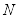Home/Class 12/Physics/

Monochromatic light of frequency $$6.0\times 10^{14}Hz$$  is produced by a laser. The power emitted is $$2.0\times 10^{-3}W$$
(a) What is the energy of a photon in the light beam?
(b) How many photons per second, on an average, are emitted by the source?Speed
00:00
02:36## QuestionPhysicsClass 12

Monochromatic light of frequency $$6.0\times 10^{14}Hz$$  is produced by a laser. The power emitted is $$2.0\times 10^{-3}W$$
(a) What is the energy of a photon in the light beam?
(b) How many photons per second, on an average, are emitted by the source?

(a) Frequency of monochromatic light, $$v=6.0\times 10^{14}Hz$$
Energy of a photon is given by;
$$E=\mathit{hv}$$
$$=6.62\times 10^{-34}\times 6.0\times 10^{14}$$
$$=3.98\times 10^{-19}$$
(b) Consider the number of photons emitted per second to be, $$N$$.
And power emitted is, . $$P=2.0\times 10^{-3}$$ $$W$$
Power transmitted in the beam equalstimes the energy per photon;
$$P=\mathit{NE}$$
$$N=\frac P E$$
$$N=\frac{2.0\times 10^{-3}}{3.98\times 10^{-19}}=5.0\times 10^{15}$$  photons per second